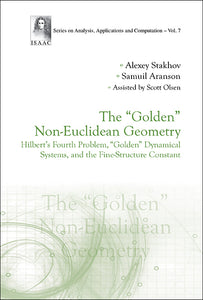# "Golden" Non-euclidean Geometry, The: Hilbert's Fourth Problem, "Golden" Dynamical Systems, And The Fine-structure Constant

Alexey Stakhov

Format: Print Book

ISBN: 9789814678292

• \$137.35
Unit price per
Tax included.

This unique book overturns our ideas about non-Euclidean geometry and the fine-structure constant, and attempts to solve long-standing mathematical problems. It describes a general theory of 'recursive' hyperbolic functions based on the 'Mathematics of Harmony,' and the 'golden,' 'silver,' and other 'metallic' proportions. Then, these theories are used to derive an original solution to Hilbert's Fourth Problem for hyperbolic and spherical geometries. On this journey, the book describes the 'golden' qualitative theory of dynamical systems based on 'metallic' proportions. Finally, it presents a solution to a Millennium Problem by developing the Fibonacci special theory of relativity as an original physical-mathematical solution for the fine-structure constant. It is intended for a wide audience who are interested in the history of mathematics, non-Euclidean geometry, Hilbert's mathematical problems, dynamical systems, and Millennium Problems.See Press Release: Application of the mathematics of harmony - Golden non-Euclidean geometry in modern math

Format: Hardcover
No of Pages: 308
Imprint: World Scientific
Publication date: 20160715
Series: Series On Analysis, Applications And Computation